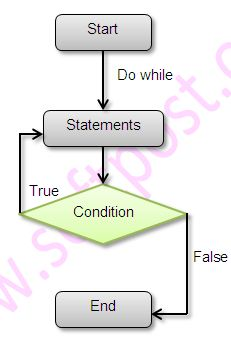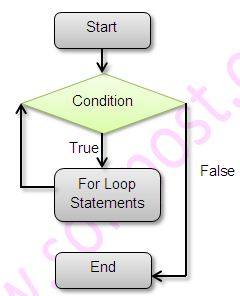There are 4 types of looping statements in Java.
• for – 2 variations
• while
• do ….while
• foreach method in Java 8 – used to iterate through collection type of class
Below example will illustrate looping statements in Java.
``````
package loops;

import java.util.ArrayList;

/**
* Created by Sagar on 16-04-2016.
*/
public class Loops {

public static void main(String [] args){
//for loop
for(int i=1;i<=3;i++){
System.out.println("Value of i in for loop -> " + i);
}

//another for loop
ArrayList<String> a = new ArrayList<>();

for(String s : a){
//Print each element in ArrayList
System.out.println("In other for loop -> " + s);
}

//do loop
int i = 1;
do{
System.out.println("Value of i in do loop -> " + i++);
}while(i<=4);

//while loop
i = 1;
while(i<=4){
System.out.println("Value of i in While loop -> " + i++);
}

//foreach using lambda expression
a.forEach((s)->System.out.println("Inside foreach loop -> " + s));

}
}
``````
Here is the output of above Java example
``````
Value of i in for loop -> 1
Value of i in for loop -> 2
Value of i in for loop -> 3
In other for loop -> Brisbane
In other for loop -> Mumbai
In other for loop -> London
Value of i in do loop -> 1
Value of i in do loop -> 2
Value of i in do loop -> 3
Value of i in do loop -> 4
Value of i in While loop -> 1
Value of i in While loop -> 2
Value of i in While loop -> 3
Value of i in While loop -> 4
Inside foreach loop -> Brisbane
Inside foreach loop -> Mumbai
Inside foreach loop -> London
``````

### break and continue keyword in Java

break keyword is used to come out of loop. continue keyword is used to jump to next iteration in a loop. These keywords can be used in for loop, while loop, do…while loop Below example illustrates the use of break and continue keywords.
``````
package loops;

/**
* Created by Sagar on 19-04-2016.
*/
public class BreakContinue {

public static void main(String [] args){

System.out.println("break keyword");
//break keyword breaks out of loop and executes next statement
for (int i=1;i<10;i++){
if (i==5)
break;
System.out.println("value of i is -> " + i);
}

System.out.println("continue keyword");
for (int i=1;i<10;i++){

//continue keyword takes control to the beginning of loop
//It does not execute statements that follow
if (i==5)
continue;
System.out.println("value of i is -> " + i);
}

int [] a = {1,2,3};
for(int v:a){
System.out.println(v);
break;
}

}
}
``````
Here is the output of above example.
``````
break keyword
value of i is -> 1
value of i is -> 2
value of i is -> 3
value of i is -> 4
continue keyword
value of i is -> 1
value of i is -> 2
value of i is -> 3
value of i is -> 4
value of i is -> 6
value of i is -> 7
value of i is -> 8
value of i is -> 9
1
``````Web development and Automation testing

solutions delivered!!